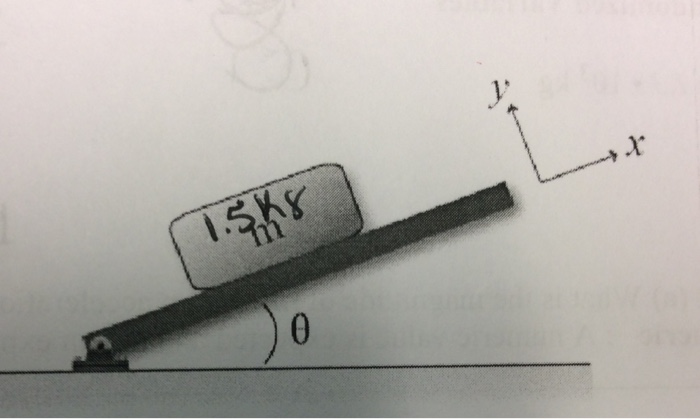# Problem: A block with a mass of m = 1.5 kg rests on a wooden plank. The coefficient of static friction between the block and the plank is μstatic = 0.86. One end of the board is attached to a hinge so that the other end can be lifted forming an angle, with respect to the ground. Assume the x-axis is along the plank as shown in figure.Determine θ in degrees if the block is on the verge of slipping.

###### FREE Expert Solution

The magnitude of the force of gravity in the y-direction is:

Fgy = - mgcosθ

The maximum frictional force is given by:

Fs = µs

But N = mgcosθ

Therefore, Fs max = µsmgcosθm

94% (254 ratings)###### Problem Details

A block with a mass of m = 1.5 kg rests on a wooden plank. The coefficient of static friction between the block and the plank is μstatic = 0.86. One end of the board is attached to a hinge so that the other end can be lifted forming an angle, with respect to the ground. Assume the x-axis is along the plank as shown in figure.

Determine θ in degrees if the block is on the verge of slipping.